﻿ 数据结构之线性表（顺序表、链表、栈、队列）

### 数据结构之线性表（顺序表、链表、栈、队列）

2021-11-28 7:41:31

### 目录

• 一、线性表
• 1.1 顺序表
• 1.1.1 顺序表的实现
• 1.1.2 顺序表的遍历（了解学习即可）
• 1.1.3 顺序表的容量可变
• 1.1.4 顺序表的时间复杂度
• 1.1.5 java中ArrayList实现（源码分析）
• 1.2 链表
• 1.2.1 单向链表
• 1.2.1.1 单向链表API设计
• 1.2.1.2 单向链表代码实现
• 1.2.2 双向链表
• 1.2.3 链表的复杂度分析
• 以下皆为链表在开发中常见的应用场景
• 1.2.4 链表（指单链表）反转
• 1.2.5 快慢指针
• 1.2.5.1 中间值问题
• 1.2.5.2 单向链表是否有环问题
• 1.2.5.3 有环链表入口问题
• 1.2.6 循环链表
• 1.2.7 约瑟夫问题
• 1.3 栈
• 1.3.1 栈概述
• 1.3.1.1 生活中的栈
• 1.3.1.2 计算机中的栈
• 1.3.2 栈的实现（本篇是以链表基准的实现方式）
• 1.3.2.1 栈API设计
• 1.3.2.2 栈代码实现
• 1.3.3 案例
• 1.3.3.1 括号匹配问题
• 1.3.3.2 逆波兰表达式求值问题
• 1.4 队列
• 1.4.1 队列的API设计
• 1.4.2 队列的实现

## 一、线性表**线性表的特征：**数据元素之间具有一种“一对一”的逻辑关系。

1. 第一个数据元素没有前驱，这个数据元素被称为头结点；
2. 最后一个数据元素没有后继，这个数据元素被称为尾结点；
3. 除了第一个和最后一个数据元素外，其他数据元素有且仅有一个前驱和一个后继。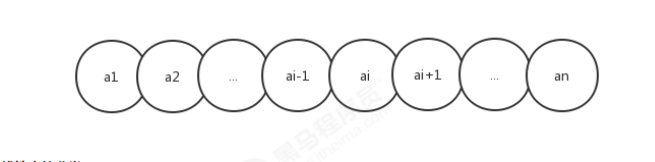### 1.1 顺序表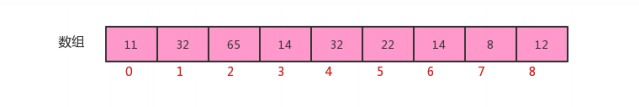#### 1.1.1 顺序表的实现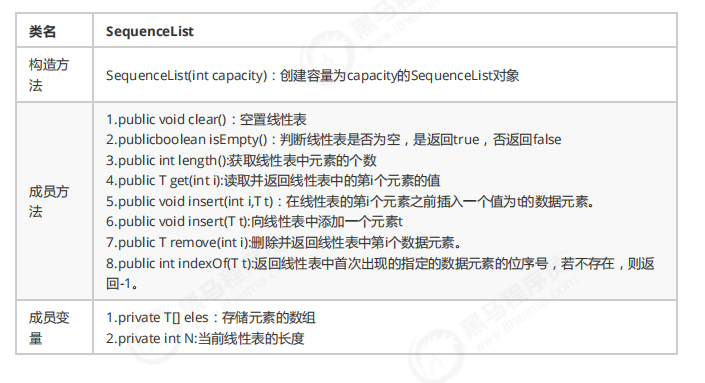``````public class SequenceList<T>{
// 存储元素的数组
private T[] eles;
// 记录当前顺序表中的元素个数
private  int N;

// 构造方法
public SequenceList(int capacity) {
// 初始化数组
this.eles = (T[])new Object[capacity];
// 初始化长度
this.N = 0;
}

// 将一个线性表置为空表
public void clear(){
this.N = 0;
}
// 判断当前线性表是否为空表
public boolean isEmpty(){
return N==0;
}
// 获取线性表的长度
public int length(){
return N;
}
// 获取指定位置的元素
public T get(int i){
return eles[i];
}
// 向线性表中添加元素t
public void insert(T t){
eles[N++] = t; // 巧妙的写法
}
//在i元素处插入元素t
public void insert(int i,T t){
// 先把i的索引处元素及其后面的元素一次向后移动一位
for (int index=N-1;index>i;index--){
eles[index] = eles[index-1];
}
// 再把他元素放到i索引处即可
eles[i] = t;
}
//删除指定位置i处的元素，并返回该元素
public T remove(int i){
// 记录索引处的值
T current = eles[i];
// 索引后面的元素依次向后移动一味即可
for (int index=i;index<N-1;index++){
eles[index] = eles[index+1];
}
// 元素个数-1
N--;
return current;
}
//查找t元素第一次出现的位置
public int indexOf(T t){
for (int i=0;i<N;i++){
if (eles[i].equals(t)) {

return 1;
}
}
return -1;
}
}
``````

#### 1.1.2 顺序表的遍历（了解学习即可）

1. 让SequenceList实现Iterable接口，重写iterator方法；
2. 在SequenceList内部提供一个内部类SIterator,实现Iterator接口，重写hasNext方法和next方法；

``````
``````

#### 1.1.3 顺序表的容量可变

1. 添加元素时：
添加元素时，应该检查当前数组的大小是否能容纳新的元素，如果不能容纳，则需要创建新的容量更大的数组，我们这里创建一个是原数组两倍容量的新数组存储元素。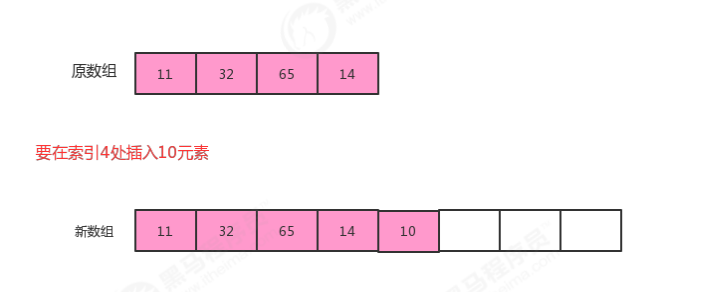2. 移除元素时：
移除元素时，应该检查当前数组的大小是否太大，比如正在用 100 个容量的数组存储 10 个元素，这样就会造成内存空间的浪费，应该创建一个容量更小的数组存储元素。如果我们发现数据元素的数量不足数组容量的1/4，则创建一个是原数组容量的1/2的新数组存储元素。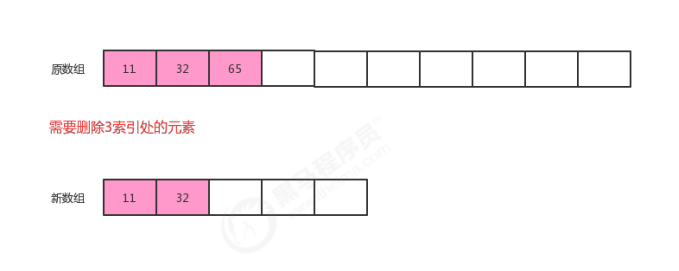``````public class SequenceList<T> implements Iterable<T>{
//存储元素的数组
private T[] eles;
//记录当前顺序表中的元素个数
private int N;

//构造方法
public SequenceList(int capacity){
//初始化数组
this.eles=(T[])new Object[capacity];
//初始化长度
this.N=0;
}

//将一个线性表置为空表
public void clear(){
this.N=0;
}

//判断当前线性表是否为空表
public boolean isEmpty(){
return N==0;
}

//获取线性表的长度
public int length(){
return N;
}

//获取指定位置的元素
public T get(int i){
return eles[i];
}

//向线型表中添加元素t
public void insert(T t){
// 扩容
if (N==eles.length){
resize(2*eles.length);
}

eles[N++]=t;
}

//在i元素处插入元素t
public void insert(int i,T t){
// 扩容
if (N==eles.length){
resize(2*eles.length);
}

//先把i索引处的元素及其后面的元素依次向后移动一位
for(int index=N;index>i;index--){
eles[index]=eles[index-1];
}
//再把t元素放到i索引处即可
eles[i]=t;

//元素个数+1
N++;
}

//删除指定位置i处的元素，并返回该元素
public T remove(int i){
//记录索引i处的值
T current = eles[i];
//索引i后面元素依次向前移动一位即可
for(int index=i;index<N-1;index++){
eles[index]=eles[index+1];
}
//元素个数-1
N--;
// 缩容
if (N<eles.length/4){
resize(eles.length/2);
}

return current;
}

//查找t元素第一次出现的位置
public int indexOf(T t){
for(int i=0;i<N;i++){
if (eles[i].equals(t)){
return i;
}
}
return -1;
}

//根据参数newSize，重置eles的大小————扩容与缩容
public void resize(int newSize){
//定义一个临时数组，指向原数组
T[] temp=eles;
//创建新数组
eles=(T[])new Object[newSize];
//把原数组的数据拷贝到新数组即可
for(int i=0;i<N;i++){
eles[i]=temp[i];
}
}
@Override
public Iterator<T> iterator() {
return new SIterator();
}

private class SIterator implements Iterator{
private int cusor;
public SIterator(){
this.cusor=0;
}
@Override
public boolean hasNext() {
return cusor<N;
}

@Override
public Object next() {
return eles[cusor++];
}
}
}
``````

#### 1.1.4 顺序表的时间复杂度

get(i):不难看出，不论数据元素量N有多大，只需要一次eles[i]就可以获取到对应的元素，所以时间复杂度为O(1);
insert(int i,T t):每一次插入，都需要把i位置后面的元素移动一次，随着元素数量N的增大，移动的元素也越多，时

remove(int i):每一次删除，都需要把i位置后面的元素移动一次，随着数据量N的增大,移动的元素也越多，时间复杂度为O(n);

#### 1.1.5 java中ArrayList实现（源码分析）

java中ArrayList集合的底层也是一种顺序表，使用数组实现，同样提供了增删改查以及扩容等功能。

1. 是否用数组实现；
2. 有没有扩容操作；
3. 有没有提供遍历方式；

### 1.2 链表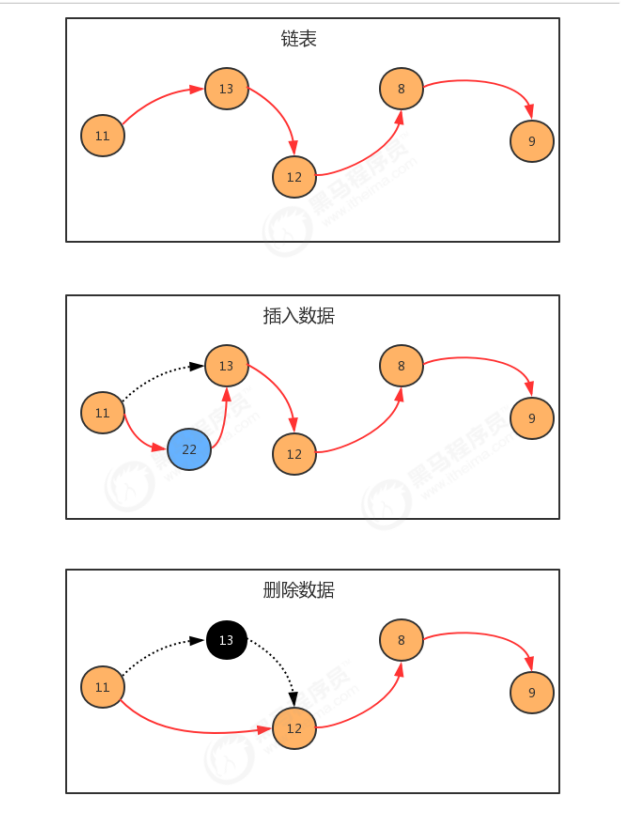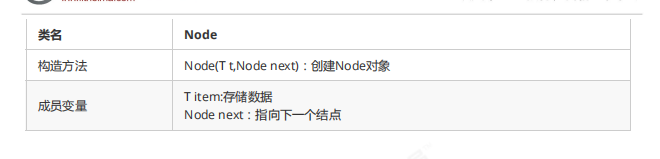``````public class Node<T> {
//存储元素
public T item;
//指向下一个结点
public Node next;
//构造方法
public Node(T item, Node next) {
his.item = item;
this.next = next;
}
}
``````

``````public static void main(String[] args) throws Exception {
//构建结点
Node<Integer> first = new Node<Integer>(11, null);
Node<Integer> second = new Node<Integer>(13, null);
Node<Integer> third = new Node<Integer>(12, null);
Node<Integer> fourth = new Node<Integer>(8, null);
Node<Integer> fifth = new Node<Integer>(9, null);
//生成链表
first.next = second;
second.next = third;
third.next = fourth;
fourth.next = fifth;
}
``````

#### 1.2.1 单向链表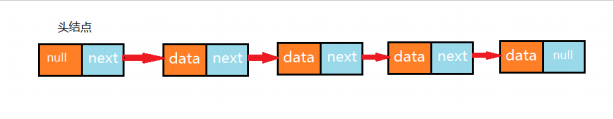##### 1.2.1.1 单向链表API设计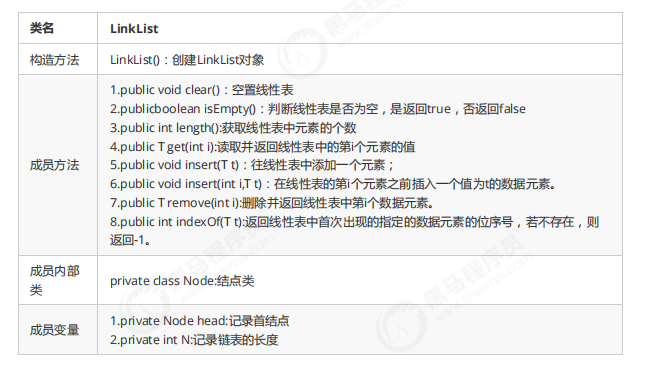##### 1.2.1.2 单向链表代码实现
``````public class LinkList<T> implements Iterable<T>{
// 记录头节点
// 记录链表的长度
private int N;

// 内部类：结点类
private class Node{
// 存储数据
T item;
// 下一个结点
Node next;

public Node(T item, Node next) {
this.item = item;
this.next = next;
}
}

// 初始化头结点
// 初始化长度
this.N = 0;
}

// 清空链表
public void clear(){
this.N=0;
}

// 获取链表的长度
public int length(){
return N;
}

// 判断链表是否为空
public boolean isEmpty(){
return N == 0;
}

// 获取指定位置i处的元素
public T get(int i){
// 通过循环，从头结点开始往后找，依次找i次，就可以找到对应的元素
for (int index=0;index<i;index++){
n= n.next;
}
return n.item;
}

// 向链表添加元素
public void insert(T t){
// 找到最后一个结点
while(n.next!=null){
n = n.next;
}
//创建新结点,保存元素t
Node newNode = new Node(t,null);
// 让当前最后一个结点指向新结点
n.next = newNode;
// 元素个数+1
N++;
}

// 向指定位置i处，添加元素
public void insert(int i,T t){
// 先找到i位置前一个结点
for (int index=0;index<=i-1;index++){
pre = pre.next;
}
// 找到i位置的结点
Node curr = pre.next;
// 创建新结点，并且新结点需要指向原来i位置的结点
Node newNode = new Node(t,curr);
// 原来i位置的前一个节点指向新结点即可
pre.next = newNode;
// 元素个数+1
N++;
}

// 删除指定位置i处的元素，并返回被删除的元素
public T remove(int i){
// 先找到i位置的前一个节点
for (int index=0;index<=i-1;index++){
pre = pre.next;
}
// 要找到i位置的结点
Node curr = pre.next;
// i位置的结点指向下一个结点,即找到i位置的下一个结点
Node newNode = curr.next;
// 前一个节点指向后一个节点
pre.next = newNode;
// 元素个数-1
N--;
return curr.item;
}
//查找元素t在链表中第一次出现的位置
public int indexOf(T t){
// 从头结点开始，一次找到每一个结点，取出item，和t比较，如果相同，就找到了
for (int i=0;n.next!=null;i++){
n = n.next;
if (n.item.equals(t)){
return i;
}
}
return -1;
}

@Override
public Iterator<T> iterator(){
return new LIterator();
}
//内部类
private class LIterator implements Iterator{
// 成员变量
private Node n;

public LIterator() {
}

@Override
public boolean hasNext() {
return n.next!=null;
}

@Override
public Object next() {
n = n.next;
return n.item;
}
}
}

``````

#### 1.2.2 双向链表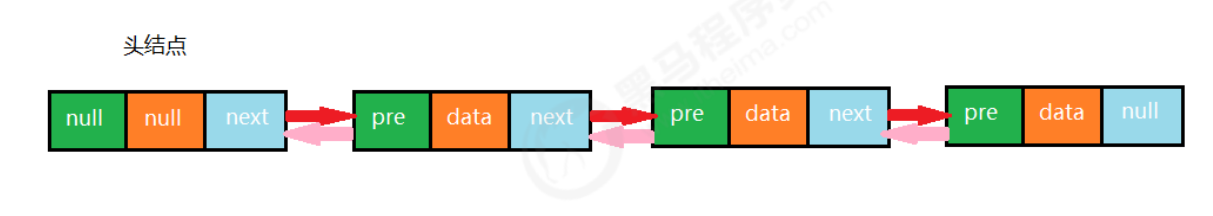1.2.2.1 结点API设计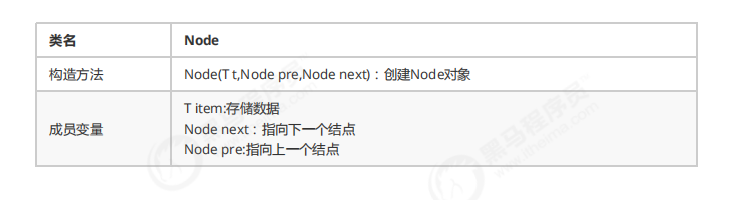1.2.2.2 双向链表API设计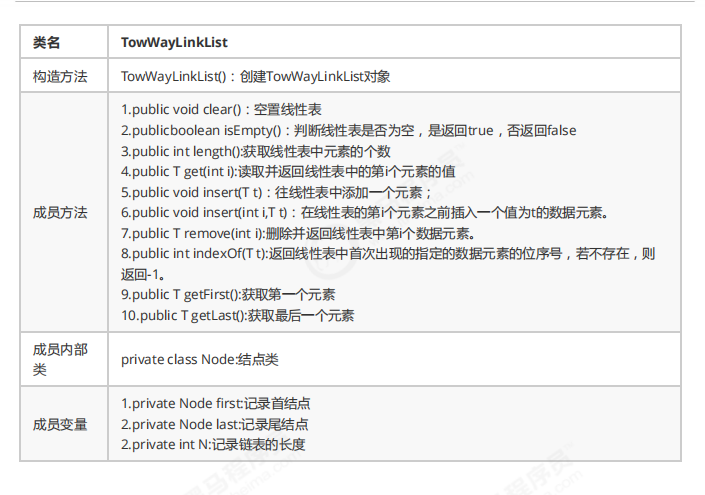1.2.2.3 双向链表代码实现

``````public class TowWayLinkList<T> implements Iterable<T> {
//首结点
//最后一个结点
private Node last;
//链表的长度
private int N;

//结点类
private class Node{
public Node(T item, Node pre, Node next) {
this.item = item;
this.pre = pre;
this.next = next;
}

//存储数据
public T item;
//指向上一个结点
public Node pre;
//指向下一个结点
public Node next;
}

//初始化头结点和尾结点
this.last=null;
//初始化元素个数
this.N=0;
}

//清空链表
public void clear(){
this.last=null;
this.N=0;
}

//获取链表长度
public int length(){
return N;
}

//判断链表是否为空
public boolean isEmpty(){
return N==0;
}

//获取第一个元素
public T getFirst(){
if (isEmpty()){
return null;
}
}

//获取最后一个元素
public T getLast(){
if (isEmpty()){
return null;
}
return last.item;
}

//插入元素t
public void insert(T t){

if (isEmpty()){
//如果链表为空：

//创建新的结点
Node newNode = new Node(t,head, null);
//让新结点称为尾结点
last=newNode;
//让头结点指向尾结点
}else {
//如果链表不为空
Node oldLast = last;

//创建新的结点
Node newNode = new Node(t, oldLast, null);

//让当前的尾结点指向新结点
oldLast.next=newNode;
//让新结点称为尾结点
last = newNode;
}
//元素个数+1
N++;
}

//向指定位置i处插入元素t
public void insert(int i,T t){
//找到i位置的前一个结点
for(int index=0;index<i;index++){
pre=pre.next;
}
//找到i位置的结点
Node curr = pre.next;
//创建新结点
Node newNode = new Node(t, pre, curr);
//让i位置的前一个结点的下一个结点变为新结点
pre.next=newNode;
//让i位置的前一个结点变为新结点
curr.pre=newNode;
//元素个数+1
N++;
}

//获取指定位置i处的元素
public T get(int i){
for(int index=0;index<i;index++){
n=n.next;
}
return n.item;
}

//找到元素t在链表中第一次出现的位置
public int indexOf(T t){
for(int i=0;n.next!=null;i++){
n=n.next;
if (n.next.equals(t)){
return i;
}
}
return -1;
}

//删除位置i处的元素，并返回该元素
public T remove(int i){
//找到i位置的前一个结点
for(int index=0;index<i;index++){
pre=pre.next;
}
//找到i位置的结点
Node curr = pre.next;
//找到i位置的下一个结点
Node nextNode= curr.next;
//让i位置的前一个结点的下一个结点变为i位置的下一个结点
pre.next=nextNode;
//让i位置的下一个结点的上一个结点变为i位置的前一个结点
nextNode.pre=pre;
//元素的个数-1
N--;
return curr.item;
}

@Override
public Iterator<T> iterator() {
return new TIterator();
}

private class TIterator implements Iterator{
private Node n;
public TIterator(){
}
@Override
public boolean hasNext() {
return n.next!=null;
}
@Override
public Object next() {
n=n.next;
return n.item;
}
}
}
``````

1. 底层是否用双向链表实现；
2. 结点类是否有三个域；

#### 1.2.3 链表的复杂度分析

get(int i):每一次查询，都需要从链表的头部开始，依次向后查找，随着数据元素N的增多，比较的元素越多，时间复杂度为O(n)

insert(int i,T t):每一次插入，需要先找到i位置的前一个元素，然后完成插入操作，随着数据元素N的增多，查找的

remove(int i):每一次移除，需要先找到i位置的前一个元素，然后完成插入操作，随着数据元素N的增多，查找的元素越多，时间复杂度为O(n)

#### 1.2.4 链表（指单链表）反转

``````public void reverse()：对整个链表反转
public Node reverse(Node curr)：反转链表中的某个结点curr,并把反转后的curr结点返回
``````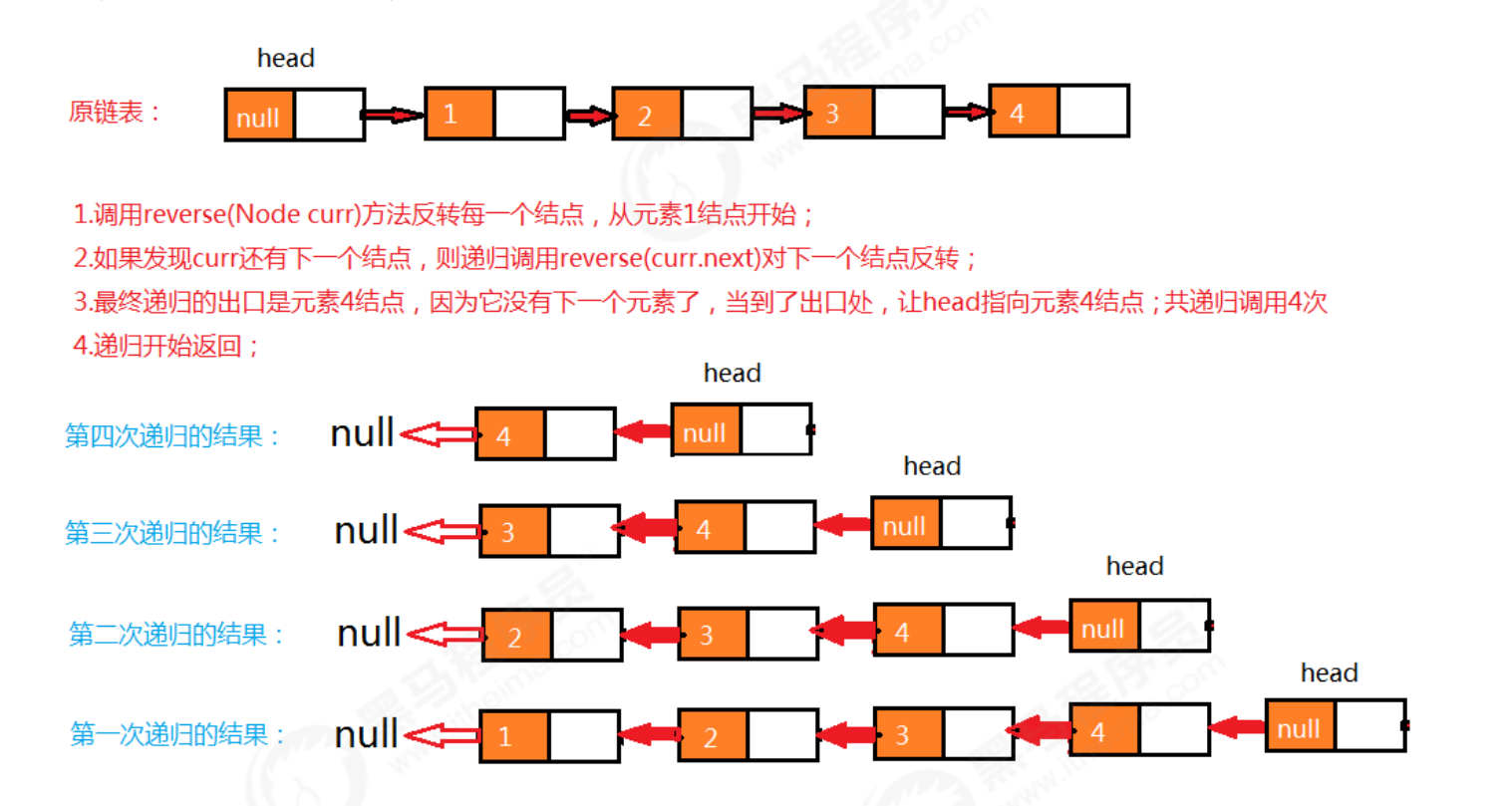``````	//用来反转整个链表
public void reverse(){

//判断当前链表是否为空链表，如果是空链表，则结束运行，如果不是，则调用重载的reverse方法完成反转
if (isEmpty()){
return;
}
}

//反转指定的结点curr，并把反转后的结点返回
public Node reverse(Node curr){
// 递归调用的出口
if (curr.next==null){
return curr;
}
//递归的反转当前结点curr的下一个结点；返回值就是链表反转后，当前结点的上一个结点
Node pre = reverse(curr.next);
//让返回的结点的下一个结点变为当前结点curr；
pre.next=curr;
//把当前结点的下一个结点变为null
curr.next=null;
return curr;
}

``````

#### 1.2.5 快慢指针

##### 1.2.5.1 中间值问题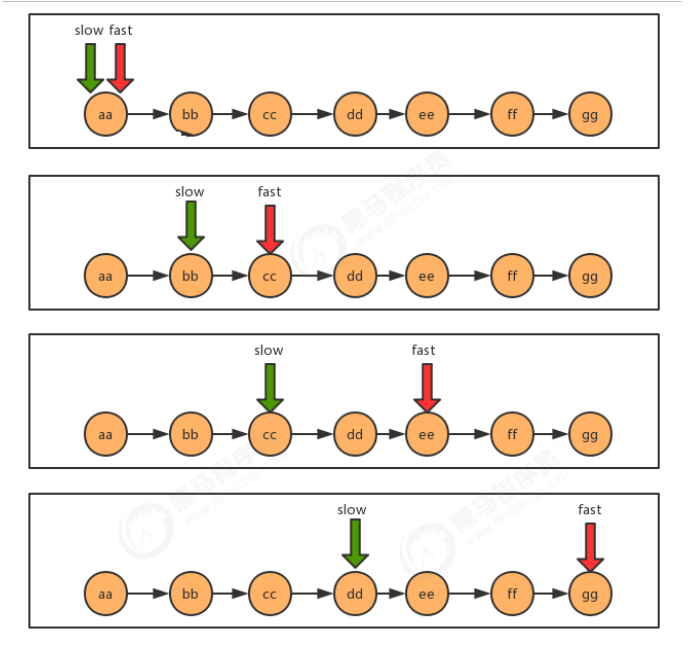``````public class FastSlowTest {

public static void main(String[] args) throws Exception {
//创建结点
Node<String> first = new Node<String>("aa", null);
Node<String> second = new Node<String>("bb", null);
Node<String> third = new Node<String>("cc", null);
Node<String> fourth = new Node<String>("dd", null);
Node<String> fifth = new Node<String>("ee", null);
Node<String> six = new Node<String>("ff", null);
Node<String> seven = new Node<String>("gg", null);

//完成结点之间的指向
first.next = second;
second.next = third;
third.next = fourth;
fourth.next = fifth;
fifth.next = six;
six.next = seven;

//查找中间值
String mid = getMid(first);
System.out.println("中间值为："+mid);
}

/**
* @param first 链表的首结点
* @return 链表的中间结点的值
*/
public static String getMid(Node<String> first) {
//定义两个指针
Node<String> fast = first;
Node<String> slow = first;
//使用两个指针遍历链表，当快指针指向的结点没有下一个结点了，就可以结束了，结束之后，慢指针指向的结点就是中间值
while(fast!=null &&fast.next!=null){
//变化fast的值和slow的值
fast = fast.next.next;
slow=slow.next;
}

return slow.item;
}

//结点类
private static class Node<T> {
//存储数据
T item;
//下一个结点
Node next;

public Node(T item, Node next) {
this.item = item;
this.next = next;
}
}
}
``````
##### 1.2.5.2 单向链表是否有环问题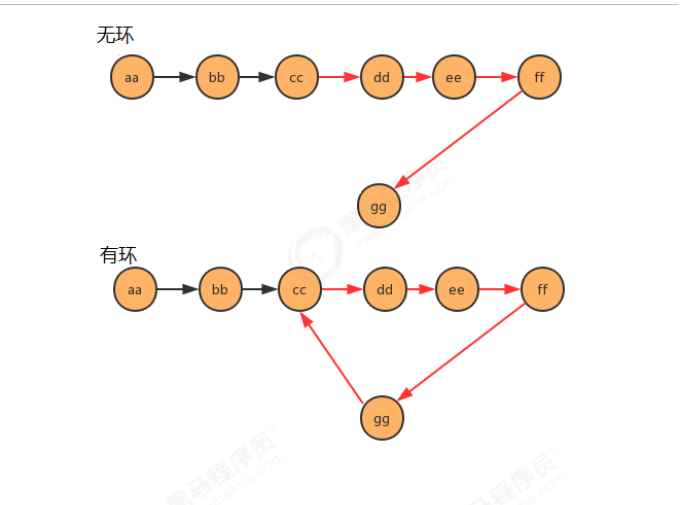``````//测试类
public class Test {
public static void main(String[] args) throws Exception {
Node<String> first = new Node<String>("aa", null);
Node<String> second = new Node<String>("bb", null);
Node<String> third = new Node<String>("cc", null);
Node<String> fourth = new Node<String>("dd", null);
Node<String> fifth = new Node<String>("ee", null);
Node<String> six = new Node<String>("ff", null);
Node<String> seven = new Node<String>("gg", null);

//完成结点之间的指向
first.next = second;
second.next = third;
third.next = fourth;
fourth.next = fifth;
fifth.next = six;
six.next = seven;
//产生环
seven.next = third;

//判断链表是否有环
boolean circle = isCircle(first);
System.out.println("first链表中是否有环："+circle);
}

/**
* 判断链表中是否有环
* @param first 链表首结点
* @return ture为有环，false为无环
*/
public static boolean isCircle(Node<String> first) {
return false;
}

//结点类
private static class Node<T> {
//存储数据 T item;
//下一个结点
Node next;
public Node(T item, Node next) {
this.item = item;
this.next = next;
}
}
}
``````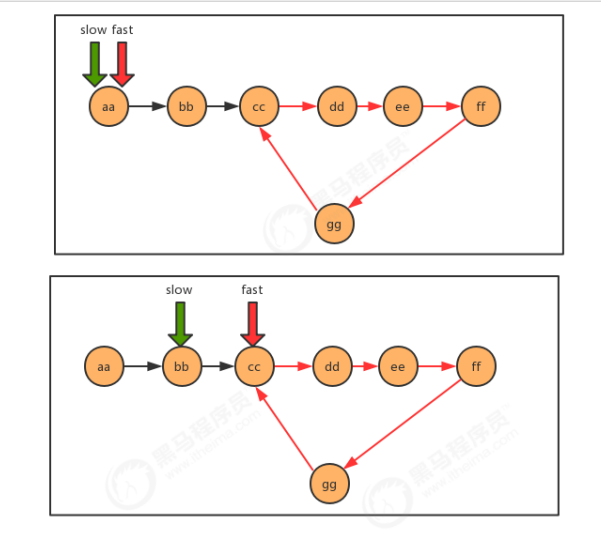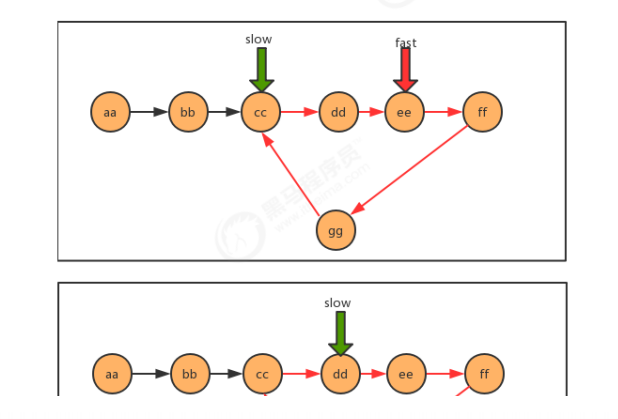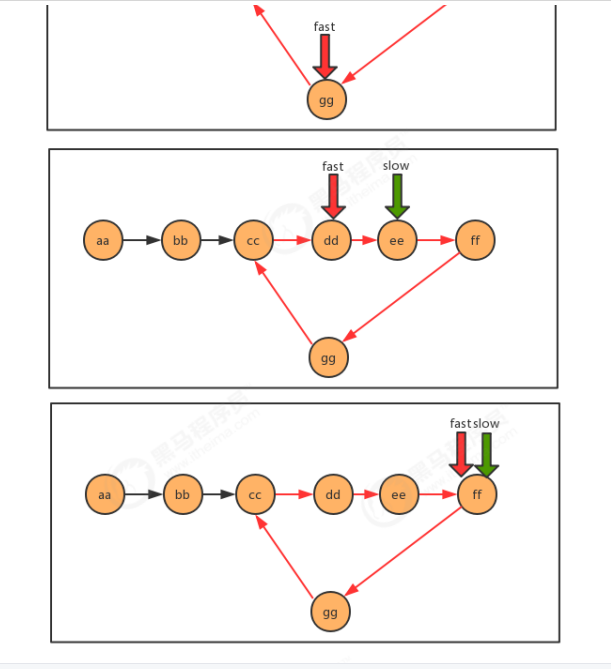``````/**
* 判断链表中是否有环
* @param first 链表首结点
* @return ture为有环，false为无环
*/
public static boolean isCircle(Node<String> first) {
//定义快慢指针
Node<String> fast = first;
Node<String> slow = first;

//遍历链表，如果快慢指针指向了同一个结点，那么证明有环
while(fast!=null && fast.next!=null){
//变换fast和slow
fast = fast.next.next;
slow = slow.next;

if (fast.equals(slow)){
return true;
}
}
return false;
}
``````
##### 1.2.5.3 有环链表入口问题

``````//测试类
public class CircleListInTest {
public static void main(String[] args) throws Exception {
Node<String> first = new Node<String>("aa", null);
Node<String> second = new Node<String>("bb", null);
Node<String> third = new Node<String>("cc", null);
Node<String> fourth = new Node<String>("dd", null);
Node<String> fifth = new Node<String>("ee", null);
Node<String> six = new Node<String>("ff", null);
Node<String> seven = new Node<String>("gg", null);

//完成结点之间的指向
first.next = second;
second.next = third;
third.next = fourth;
fourth.next = fifth;
fifth.next = six;
six.next = seven;
//产生环
seven.next = third;

//查找环的入口结点
Node<String> entrance = getEntrance(first);
System.out.println("first链表中环的入口结点元素为："+entrance.item);
}
``````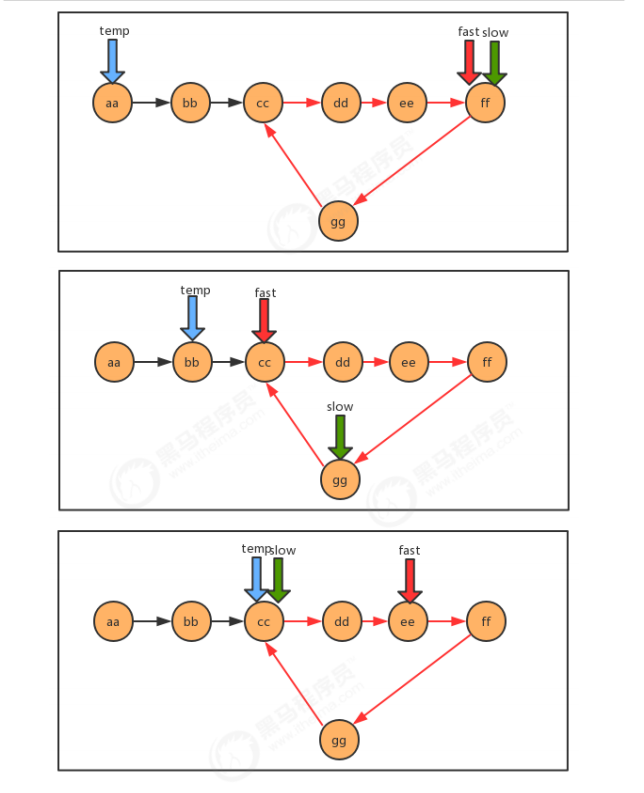``````/**
* 查找有环链表中环的入口结点
* @param first 链表首结点
* @return 环的入口结点
*/
public static Node getEntrance(Node<String> first) {
//定义快慢指针
Node<String> fast = first;
Node<String> slow = first;
Node<String> temp = null;

//遍历链表，先找到环(快慢指针相遇),准备一个临时指针，指向链表的首结点，继续遍历，直到慢指针和临时指针相遇，那么相遇时所指向的结点就是环的入口
while(fast!=null && fast.next!=null){
//变换快慢指针
fast = fast.next.next;
slow = slow.next;

//判断快慢指针是否相遇
if (fast.equals(slow)){
temp = first;
continue;
}

//让临时结点变换
if (temp!=null){
temp = temp.next;
//判断临时指针是否和慢指针相遇
if (temp.equals(slow)){
break;
}
}
}
return temp;
}
``````

#### 1.2.6 循环链表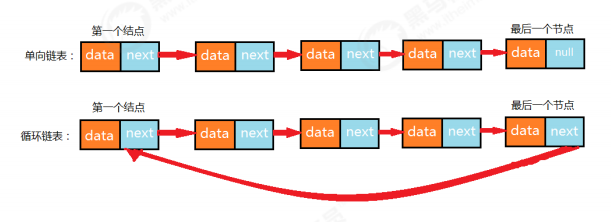#### 1.2.7 约瑟夫问题

41 个人坐一圈，第一个人编号为 1 ，第二个人编号为 2 ，第n个人编号为n。

1. 编号为 1 的人开始从 1 报数，依次向后，报数为 3 的那个人退出圈；
2. 自退出那个人开始的下一个人再次从 1 开始报数，以此类推；
3. 求出最后退出的那个人的编号。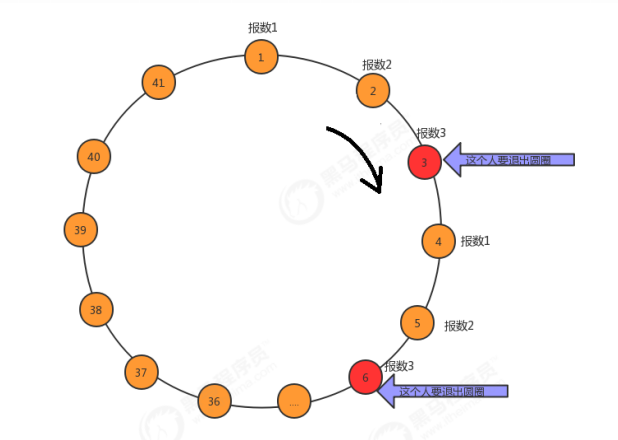1. 构建含有 41 个结点的单向循环链表，分别存储1~41的值，分别代表这 41 个人；
2. 使用计数器count，记录当前报数的值；
3. 遍历链表，每循环一次，count++；
4. 判断count的值，如果是 3 ，则从链表中删除这个结点并打印结点的值，把count重置为 0 ；

``````public class JosephTest {
public static void main(String[] args) {
//解决约瑟夫问题

//1.构建循环链表，包含41个结点，分别存储1~41之间的值
//用来就首结点
Node<Integer> first = null;
//用来记录前一个结点
Node<Integer> pre = null;

for(int i = 1;i<=41;i++){

//如果是第一个结点
if (i==1){
first = new Node<>(i,null);
pre = first;
continue;
}

//如果不是第一个结点
Node<Integer> newNode = new Node<>(i, null);
pre.next=newNode;
pre=newNode; // 重置pre
//如果是最后一个结点，那么需要让最后一个结点的下一个结点变为first,变为循环链表了
if (i==41){
pre.next=first;
}

}

//2.需要count计数器，模拟报数
int count=0;
//3.遍历循环链表
//记录每次遍历拿到的结点，默认从首结点开始
Node<Integer> n = first;
//记录当前结点的上一个结点
Node<Integer> before = null;
while(n!=n.next){ // 当不是自环的时候
//模拟报数
count++;
//判断当前报数是不是为3
if (count==3){
//如果是3，则把当前结点删除调用，打印当前结点，重置count=0，让当前结点n后移
before.next=n.next;
System.out.print(n.item+",");
count=0;
n=n.next;
}else{
//如果不是3，让before变为当前结点，让当前结点后移；
before=n;
n=n.next;
}
}

//打印最后一个元素
System.out.println(n.item);
}

//结点类
private static class Node<T> {
//存储数据
T item;
//下一个结点
Node next;

public Node(T item, Node next) {
this.item = item;
this.next = next;
}
}
}
``````

### 1.3 栈

#### 1.3.1 栈概述

##### 1.3.1.1 生活中的栈##### 1.3.1.2 计算机中的栈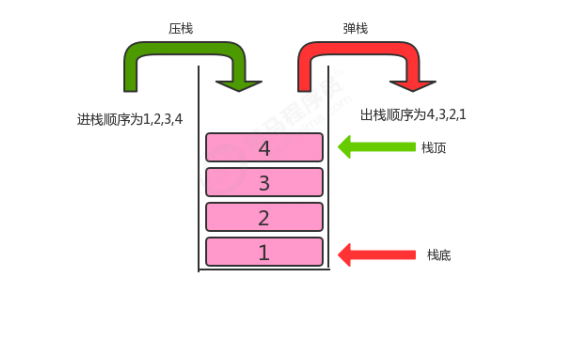#### 1.3.2 栈的实现（本篇是以链表基准的实现方式）

（注意：栈是一种逻辑层面的数据结构，因为数据的存储方式为物理存储，即顺序存储结构（数组）和链式存储结构（链表）。所以栈的实现有两种，即数组实现和链表实现）

##### 1.3.2.1 栈API设计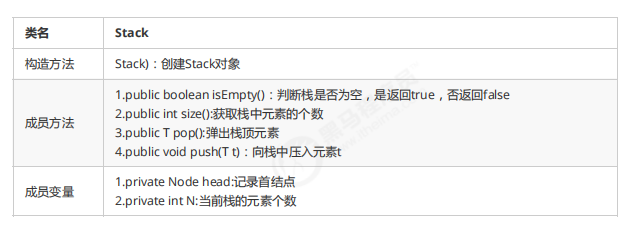##### 1.3.2.2 栈代码实现
``````public class Stack<T> implements Iterable<T>{
//记录首结点
//栈中元素的个数
private int N;

private class Node{
public T item;
public Node next;

public Node(T item, Node next) {
this.item = item;
this.next = next;
}
}

public Stack() {
this.N=0;
}

//判断当前栈中元素个数是否为0
public boolean isEmpty(){
return N==0;
}

//获取栈中元素的个数
public int size(){
return N;
}

//把t元素压入栈
public void push(T t){
//找到首结点指向的第一个结点
//创建新结点
Node newNode = new Node(t, null);
//让首结点指向新结点
//让新结点指向原来的第一个结点
newNode.next=oldFirst;
//元素个数+1；
N++;
}

//弹出栈顶元素
public T pop(){
//找到首结点指向的第一个结点
if (oldFirst==null){
return null;
}
//让首结点指向原来第一个结点的下一个结点
//元素个数-1；
N--;
return oldFirst.item;
}

@Override
public Iterator<T> iterator() {
return new SIterator();
}

private class SIterator implements Iterator{
private Node n;

public SIterator(){
}

@Override
public boolean hasNext() {
return n.next!=null;
}

@Override
public Object next() {
n = n.next;
return n.item;
}
}
}
// 测试类
public class StackTest {
public static void main(String[] args) {
//创建栈对象
Stack<String> stack = new Stack<>();

//测试压栈
stack.push("a");
stack.push("b");
stack.push("c");
stack.push("d");

for (String item : stack) {
System.out.println(item);
}
System.out.println("------------------------------");
//测试弹栈
String result = stack.pop();
System.out.println("弹出的元素是："+result);
System.out.println("剩余的元素个数："+stack.size());

}
}

``````

#### 1.3.3 案例

##### 1.3.3.1 括号匹配问题

``````给定一个字符串，里边可能包含"()"小括号和其他字符，请编写程序检查该字符串的中的小括号是否成对出现。

"(上海)(长安)"：正确匹配
"上海((长安))"：正确匹配
"上海(长安(北京)(深圳)南京)":正确匹配
"上海(长安))"：错误匹配
"((上海)长安"：错误匹配
``````

1. 创建一个栈用来存储左括号
2. 从左往右遍历字符串，拿到每一个字符
3. 判断该字符是不是左括号，如果是，放入栈中存储
4. 判断该字符是不是右括号，如果不是，继续下一次循环
5. 如果该字符是右括号，则从栈中弹出一个元素t；
6. 判断元素t是否为null，如果不是，则证明有对应的左括号，如果不是，则证明没有对应的左括号
7. 循环结束后，判断栈中还有没有剩余的左括号，如果有，则不匹配，如果没有，则匹配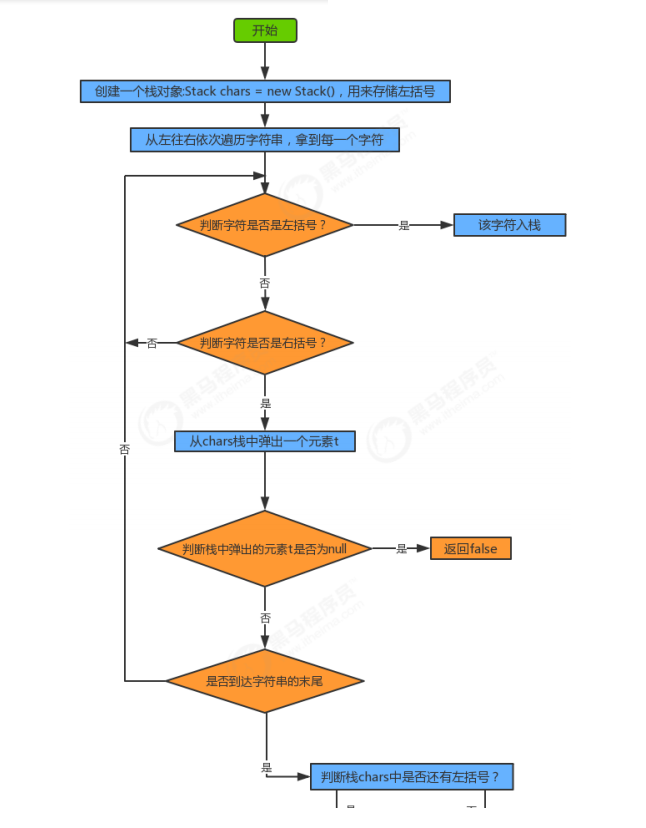代码实现：
``````public class BracketsMatchTest {
public static void main(String[] args) {
String str = "上海(长安)())";
boolean match = isMatch(str);
System.out.println(str+"中的括号是否匹配："+match);
}

/**
* 判断str中的括号是否匹配
* @param str 括号组成的字符串
* @return 如果匹配，返回true，如果不匹配，返回false
*/
public static boolean isMatch(String str){
//1.创建栈对象，用来存储左括号
Stack<String> chars = new Stack<>();
//2.从左往右遍历字符串
for (int i = 0; i < str.length(); i++) {
String currChar = str.charAt(i)+ "";
//3.判断当前字符是否为左括号，如果是，则把字符放入到栈中
if (currChar.equals("(")){
chars.push(currChar);
}else if(currChar.equals(")")){
//4.继续判断当前字符是否是有括号，如果是，则从栈中弹出一个左括号，并判断弹出的结果是否为null,如果为null证明没有匹配的左括号，如果不为null，则证明有匹配的左括号
String pop = chars.pop();
if (pop==null){
return false;
}
}
}
//5.判断栈中还有没有剩余的左括号，如果有，则证明括号不匹配
if (chars.size()==0){
return true;
}else{
return false;
}
}
}
``````
##### 1.3.3.2 逆波兰表达式求值问题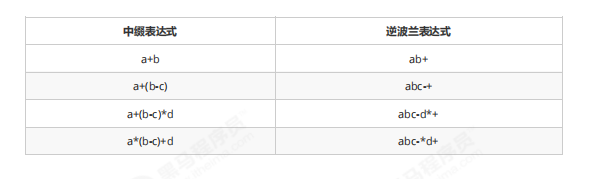``````public class ReversePolishNotation {
public static void main(String[] args) {
//中缀表达式3*（17-15）+18/6的逆波兰表达式如下
String[] notation = {"3", "17", "15", "-", "*","18", "6","/","+"};
int result = caculate(notation);
System.out.println("逆波兰表达式的结果为："+result);
}
/**
* @param notaion 逆波兰表达式的数组表示方式
*  @return 逆波兰表达式的计算结果
*/
public static int caculate(String[] notaion){
return -1;
}
}
``````

1. 创建一个栈对象oprands存储操作数
2. 从左往右遍历逆波兰表达式，得到每一个字符串
3. 判断该字符串是不是运算符，如果不是，把该该操作数压入oprands栈中
4. 如果是运算符，则从oprands栈中弹出两个操作数o 1 ,o 2
5. 使用该运算符计算o 1 和o 2 ，得到结果result
6. 把该结果压入oprands栈中
7. 遍历结束后，拿出栈中最终的结果返回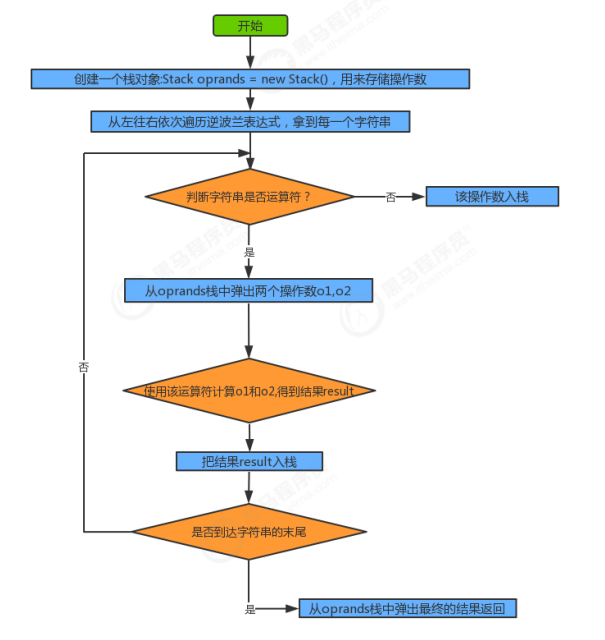代码实现：
``````public class ReversePolishNotationTest {
public static void main(String[] args) {
//中缀表达式 3*（17-15）+18/6 的逆波兰表达式如下 6+3=9
String[] notation = {"3", "17", "15", "-", "*", "18", "6", "/", "+"};
int result = caculate(notation);
System.out.println("逆波兰表达式的结果为：" + result);
}

/**
* @param notaion 逆波兰表达式的数组表示方式
* @return 逆波兰表达式的计算结果
*/
public static int caculate(String[] notaion) {
//1.定义一个栈，用来存储操作数
Stack<Integer> oprands = new Stack<>();
//2.从左往右遍历逆波兰表达式，得到每一个元素
for (int i = 0; i < notaion.length; i++) {
String curr = notaion[i];
//3.判断当前元素是运算符还是操作数
Integer o1;
Integer o2;
Integer result;
switch (curr) {
case "+":
//4.运算符，从栈中弹出两个操作数，完成运算，运算完的结果再压入栈中
o1 = oprands.pop();
o2 = oprands.pop();
result = o2 + o1;
oprands.push(result);
break;
case "-":
//4.运算符，从栈中弹出两个操作数，完成运算，运算完的结果再压入栈中
o1 = oprands.pop();
o2 = oprands.pop();
result = o2 - o1;
oprands.push(result);
break;
case "*":
//4.运算符，从栈中弹出两个操作数，完成运算，运算完的结果再压入栈中
o1 = oprands.pop();
o2 = oprands.pop();
result = o2 * o1;
oprands.push(result);
break;
case "/":
//4.运算符，从栈中弹出两个操作数，完成运算，运算完的结果再压入栈中
o1 = oprands.pop();
o2 = oprands.pop();
result = o2 / o1;
oprands.push(result);

break;
default:
//5.操作数，把该操作数放入到栈中；
oprands.push(Integer.parseInt(curr));
break;
}
}

//6.得到栈中最后一个元素，就是逆波兰表达式的结果
int result = oprands.pop();

return result;
}
}
``````

### 1.4 队列

（如同栈的实现方式，本篇以链表的方式实现）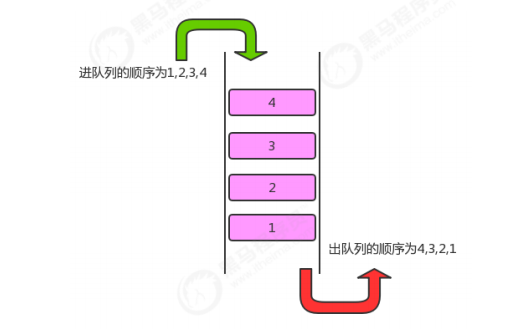#### 1.4.1 队列的API设计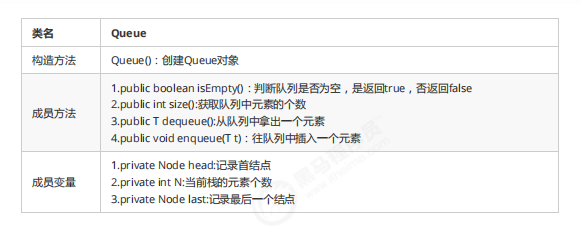#### 1.4.2 队列的实现

``````public class Queue<T> implements Iterable<T>{
//记录首结点
//记录最后一个结点
private Node last;
//记录队列中元素的个数
private int N;

private class Node{
public T item;
public Node next;

public Node(T item, Node next) {
this.item = item;
this.next = next;
}
}
public Queue() {
this.last=null;
this.N=0;
}

//判断队列是否为空
public boolean isEmpty(){
return N==0;
}

//返回队列中元素的个数
public int size(){
return N;
}

//向队列中插入元素t
public void enqueue(T t){

if (last==null){
//当前尾结点last为null
last= new Node(t,null);
}else {
//当前尾结点last不为null
Node oldLast = last;
last = new Node(t, null);
oldLast.next=last;
}

//元素个数+1
N++;
}

//从队列中拿出一个元素
public T dequeue(){
if (isEmpty()){
return null;
}

N--;

//因为出队列其实是在删除元素，因此如果队列中的元素被删除完了，需要重置last=null;

if (isEmpty()){
last=null;
}
return oldFirst.item;
}

@Override
public Iterator<T> iterator() {
return new QIterator();
}

private class QIterator implements Iterator{
private Node n;

public QIterator(){
}
@Override
public boolean hasNext() {
return n.next!=null;
}

@Override
public Object next() {
n = n.next;
return n.item;
}
}
}
``````